# Kerala Syllabus 10th Standard Chemistry Solutions Chapter 7 Chemical Reactions of Organic Compounds

You can Download Chemical Reactions of Organic Compounds Questions and Answers, Summary, Activity, Notes, Kerala Syllabus 10th Standard Chemistry Solutions Chapter 7 help you to revise complete Syllabus and score more marks in your examinations.

## Kerala State Syllabus 10th Standard Chemistry Solutions Chapter 7 Chemical Reactions of Organic Compounds

### Chemical Reactions of Organic Compounds Text Book Questions and Answers

Chapter 7 Chemistry Question 1.
Complete stages 2, 3 and 4 in the respective order.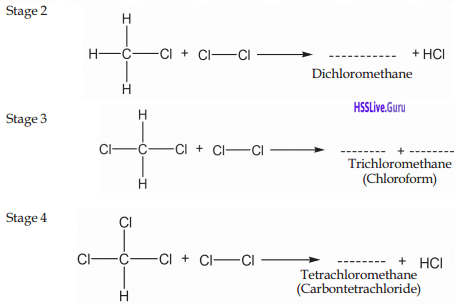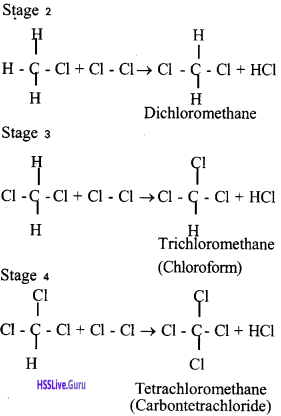Organic Chemistry Class 10 SCERT Question 2.
What are the compounds formed when CH3-CH3 (ethane) undergoes substitution reaction with chlorine? Write them.
CH3 – CH2 Cl, CH3 – CHCl2,
CH3 – CCl3, CH2Cl – CCl3,
CHCl3 – CCl3, CCl3 – CCl3Chapter 7 Chemical Reactions Question 3.
Write down the structural formulae of ethane and ethene.
CH3 – CH3 – Ethane
CH2 = CH2 – Ethene

Methyl Propanoate Question 4.
What is the peculiarity of the Carbon- Carbon bond in ethene?
In ethene, There is carbon – carbon double bond

Text Book Page No: 121

Question 5.
What do we get as the product ?
Ethane

Question 6.
Which hydrocarbon is the reactant here? ………..
Unsaturated propene

Ethene Structural Formula Question 7.
Is the product saturated or unsaturated ?
Saturated Compounds

Question 8.
Complete table 7.1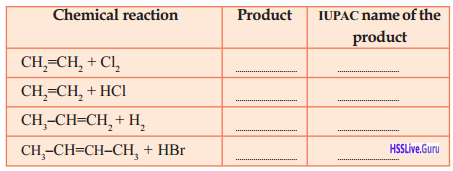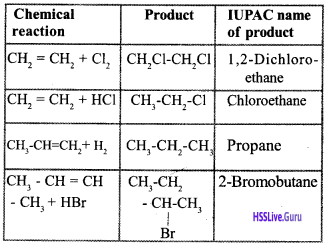Text Book Page No: 123

Substitute of Polyvinyl Alcohol Question 9.
Complete table 7.2 Suitably.Monomer Polymer Uses Vinyl Chloride PVC Pipe, Helmet Ethene Polyethane Carry bags Isoprene Natural rubber (Poly Isoprene) Tire Tetra Fluro ethene Teflon Nonstick pan

Text Book Page No: 124

Daily Chemicals Question 10.
Can you write the balanced chemical equation for the combustion of the fuel butane (C4H10) ?
2 C4H10(g) + 13O2(g) → 8CO2 + 10H2O + heat

Text Book Page No: 125

Question 11.
Complete Table 7.3 and 7.4 contain¬ing chemical reactions of hydrocarbons.Question 12.
Match Columns A, B, and C suitably.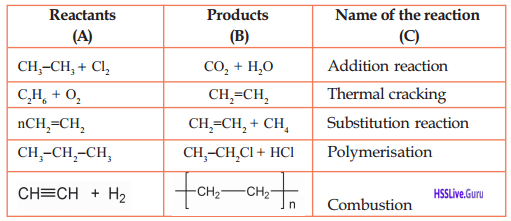Reactants (A) Products (B) Name of Reaction (C) CH3 – CH3 + Cl2 CH3 – CH2 Cl + HCl Substitution Reaction C2H6+O2 CO2 + H2O Combustion n CH2 = CH2 [CH2 – CH2]n Polymerisation CH3– CH2 – CH3 CH2 = CH2 + CH4 Thermal Cracking CH = CH + H2 CH2 = CH2 Addition Reaction

Question 13.
CH2 – OH, CH3 – CH2 – OH
Can you write the IUPAC names of these two compounds?
CH3 – OH – Methanol
CH3 – CH2 – OH – Ethanol

Text Book Page No: 126

Question 14.
Complete the following word web including more uses of ethanol.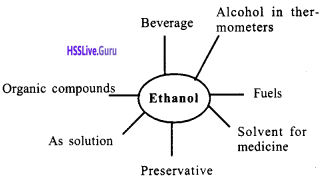Question 15.
List out the uses of ethanoic acid.

• In the manufacture of rayon
• In the rubber and silk industry.
• Vinegar – Impart Sour taste for food item.
• Used as preservative.

Text Book Page No: 129

Question 16.
Examine the given structural formulae and select the esters. You may also identify the chemicals required for their preparation.
1. CH3 – CH2 – COO – CH3
2. CH3 – CH2 – COOH
3. CH3 – CH2 – CO – CH3
4. CH3 – OH
5. CH3 – CH2 – CH2OH
6. CH3 – COOH
7. CH3 – COO – CH2 – CH2 – CH3
1. CH3 – CH2 – COO – CH3
7. CH3 – COO – CH2 – CH2 – CH3 are esters
1. CH3 – CH2 – COO – CH3
CH3 – CH2 – COOH + OH – CH3 $$\frac{\mathrm{Conc} . \mathrm{H}_{2} \mathrm{SO}_{4}}{ }$$ CH3 – CH2 – COO – CH3+ H2O
7. CH3 – COO – CH2 – CH2 – CH3
CH3 – COOH + OH – CH2 – CH2 – CH3 $$\frac{\mathrm{Conc} . \mathrm{H}_{2} \mathrm{SO}_{4}}{ }$$ CH3 – COO – CH2 – CH2 – CH3 + H2O

Text Book Page No: 130

Question 17.
Take 10 mL distilled water in a test tube and take the same volume of hard water in another test tube. Add a few drops of soap solution to both the test tubes and shake well. Do both the test tubes contain the same quantity of foam? Which test tube contains more foam? What do you infer?
No. Both the test tube does not contain same quantity of foam. Distilled water taken test tube contains more foam. Soap does not lather well in hard water. The hardness of water is due to dissolved calcium and magnesium salts in it. These salts react with soap to form insoluble compounds resulting in the decrease of lather.

Question 18.
Take 10 mL each of hard water in two test tubes. Add a few drops of soap solution in the first test tube and add the same amount of detergent solution in the second one. Shake both the test tubes well. What do you observe? Which test tube contains more foam?
Soap does not lather well in hard water. The hardness of water is due to dissolved calcium and magnesium salts in it. These salts react with soap to form insoluble compounds resulting in the decrease of lather. But detergents do not give insoluble components on reaction with these salts. Hence detergents are more effective than soaps in hard water.Question 19.
List out the merits and demerits of detergents, compared to soaps.
Merit:
Detergents are more effective than soaps in hard water.
Detergents are effective in acidic solutions.
Demerits:
excessive use of the detergents causes environmental problems. The microorganisms in water cannot decompose the components of detergents. Hence the detergents released into water lead to the destruction of aquatic life. For example, the detergents which contain phosphate increases the growth of algae and limits the quantity of oxygen. Therefore, it dej creases the quantity of oxygen for the breath of the organisms in water and causes their destruction.

Let Us Assess

Question 1.
Given below are two chemical equations.
a. CH2 = CH2 + H2 → A
b. $$\mathrm{A}+\mathrm{Cl}_{2} \quad \stackrel{\text { sunlight }}{\longrightarrow} \mathrm{B}+\mathrm{HCl}$$
Identify the compounds A and B. Name these reactions.Question 2.
Name the important chemical reactions of hydrocarbons. Give one example for each.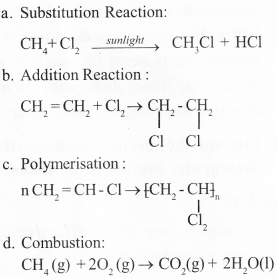e. Thermal cracking:
CH3 – CH2 – CH2 -CH3 → CH2 = CH2 + CH3 – CH3

Question 3.
Write chemical formula of propane. Write the names and structural formulae of two compounds, that may be formed during its substitution reaction with chlorine.
CH3 – CH3 – CH3 Propane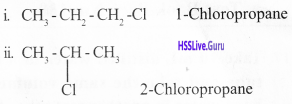Question 4.
Complete the equation for the following chemical reaction. Name this reaction.
$$\mathrm{CH}_{3}-\mathrm{CH}_{2}-\mathrm{CH}_{2}-\mathrm{CH}_{3}+\ldots \ldots \ldots \ldots \mathrm{O}_{2} \rightarrow–\mathrm{-}+$$
CH3 – CH2 – CH2 – CH3 + 13/2 O2
→ 4CO2 + 5 H2O , Combustion.

Question 5.
Which of the given molecules can form po¬lymers ? Butane, Propane, Propane, Methane, Butene.
Propene, Butene

Extended Activities

Question 1.
You are familiar with different chemical reactions of hydrocarbons. Identify the situations in daily life in which these are used.
a. Substitution Reaction : Chloroform, CCl4 preparation
b. Addition Reaction : Conversion of unsaturated compounds into saturated.
c. Combustion: Preparation of polymers like PVC.
d. Thermal Cracking: Butane (LPG)can be prepared from higher hydrocarbons

Question 2.
List out the different uses of ethanol. Pre-pare an essay on its adverse effects on human body and the related social issues when it is used as a beverage.
Uses of ethanol :

• Fuels
• Medicines
• Preservatives
• Preparation of organic compounds

Health problems :

• Reason for aneamia .
• Increases possibility of cancer
• Problems related with heart

Social problems:

• Reason for spoiling family relationships
• May cause financial crisis

Question 3.
You know how to make soap, don’t you? Try to prepare soaps of different colors and fragrance.Prepare a short note on chemistry of soaps.
Fats and oils are esters. They react with alkalies such as NaOH, KOH to form Sodium/ Potassium Salts of their carboxylic acids and glycerol.ester formed from fatty acids + NaOH / KOH → Soap + glycerol. Fatty acids such as palmitic acid, stearic acid react with alcohol, glycerol to form esters. Oils and fats are esters formed by the reaction between glycerol with fatty acid and stearic acid. Soaps are the salts formed when these react with alkalies.

### Chemical Reactions of Organic Compounds Orukkam Questions and Answers

Question 1.
After completing the chemical reactions write down to which category they belong.
a. CH2Cl + Cl2 → …….. + HCl
b. CH = CH+H2 →…………
c. CH4 + 2O2 → …….. + H2O
d. CH3 – CH2 – CH3 → ……….
a. CH2Cl + Cl2 → CH2Cl2 + HCl Substitution reaction
b. CH = CH + H2 → CH2 = CH2 Addition Reaction
c. CH4 + 2O2 → CO2 + H2O Combustion
d. CH3 – CH2 – CH3 → CH2 = CH2 + CH4 Thermal cracking

Question 2.
Rearrange the table suitably.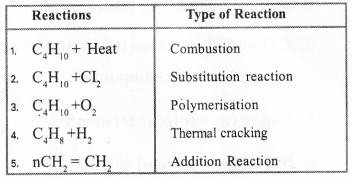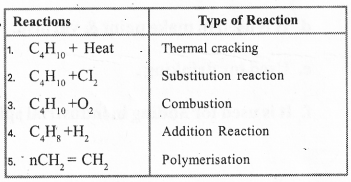Question 3.
Methane is reacting with Cl in presence of sunlight. Complete equation of that reaction.a. Write down the reaction of C3H8 with chlorine.
b. What type of reaction is this?C3HCl7+Cl2 → C3Cl8 + HCl
b. Subsititution Reaction

Question 4.
Examples of additon reaction are given below, complete the equation.
a. CH2 = CH2 + H2 → ………
b. CH2 = CH + Cl2 → ……..
c. CH = CH + H2 → ……….
d. CH = CH + Cl2→ ………Question 5.
a. Examples for combination of Hydrocarbon are given below complete the equation and balance it.
CH4 + O2 → ……. + ……….
C2H6 + O2 → ……. + ………
C3H8 + O2 → …….. + ………
b. Products formed on combustion of Hydrocarbon are ………….
a. CH4 + O2 → CO2 + 2H2O
2C2H6 + 7O2 → 4CO2 + 6H2O
C3H8 + 5O2 → 3CO2 + 4H2O
b. Carbon dioxide and WaterQuestion 6.
a. Name the product and what type of reaction is this?
nCH2 = CHCl → ………..
b. Write down the names of monomer in it.
c. Give examples for natural polymers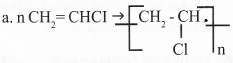Polyvinyl chloride, polymerization
b. Vinyl chloride
c. Polyisoprene, Protein

Question 7.
Complete the table.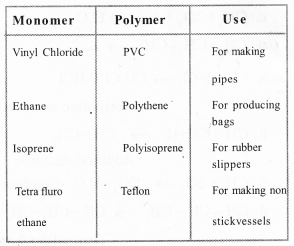Question 8.
Arrange the points in two separate columns write column heading also.
a. CO and H2 are reacted in presence of a catalyst to form compound.
b. Sugar cane juice is fermented.
c. It is known as wood spirit.
d. It is used to make paint & varnish.
e. Used for drinking.
f. It is used for adding in industrial spirit.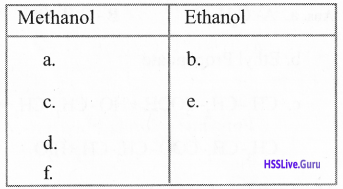Question 9.
a. Ethanol has very large industrial utility. When it enter into our body it creates large amount of problems in our body as well as in our society. List out the probl-em happening in our body and in the society.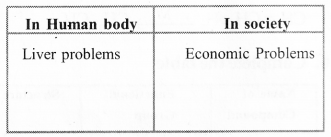b. In industrial ethanol always Methanol is added to prevent misuse by humans. Name the process and what are the side effects formed after consuming it?
a.

 In human body In society Liver problems Economic problems Cliolestrol Family issues Kidney problems Loses personality

b. Denatured Spirit:

• Loses eyesight permanently
• Vomiting

### Chemical Reactions of Organic Compounds SCERT Questions and Answers

Question 10.
Analyze the reactions and answer the following questions.a. Identify A, B.
b. Write the name of the compound ‘a’.
c. Write the name of the reaction by which ‘b’ is formed.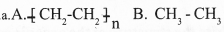b. Polythene

Question 11.
Some reactions of propane are given.
i. Hydrogen atoms are substituted one by one, in presence of sunlight.
ii. When heated in the absence of air, it decomposes to hydrocarbons with lesser molecular mass.
iii. Combines with oxygen to give C02 and H2O.
a. Identify the type of reaction in each case.
b. Write the chemical equation of the reaction (ii).
a. i. Substitutional reaction
ii. Thermal cracking
iii. Combustion
b. CH3 – CH2 – CH3 → CH2 = CH2 + CH4

Question 12.
Analyse the reactions and answer the following questions.
$$\text { i. } \mathrm{CH}_{3}-\mathrm{OH}+\mathrm{CO} \stackrel{\text { Catalyst }}{\longrightarrow} \ldots \mathrm{A}$$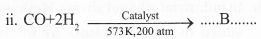$$\text { iii. } \mathrm{A}+\mathrm{B} \stackrel{\mathrm{Con} . \mathrm{H}_{2} \mathrm{SO}_{4}}{\longrightarrow} \quad \ldots . \mathrm{C} \ldots .+\mathrm{H}_{2} \mathrm{O}$$
a. Identify A, B, C.
b. What is the general name/ class to which product ‘C’ belongs? Write the IUPAC name.
a. A — CH3 – COOH
B – Methanol/CH3 – OH
C – CH3 – COO – CH3
b. Esters, Methyl ethanoate

Question 13.
Analyze the given reactions and answer the following questions.a. Identify A and B
b. What is the name of reaction by which ‘B’ is formed?
a. A – CH2 = CH2, B – CH3 Cl
b. Substitution reaction

Question 14.
Some reactions regarding the production of ethanol are given below.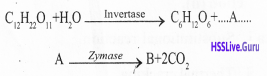a. Identify A and B.
b. Write the name of the ester formed when the product B reacts with propanoic acid,
c. Write the chemical equation for the formation of the ester.
a. A- C6 H12 O6 B – C2H5 – OH
b. Ethyl Propanoate
c. CH3 – CH2 – COOH + HO – CH2 – CH3
CH3 – CH2 – COO – CH2 – CH3 + H2O

Question 15.
Acetylene (ethyne) is prepared in the laboratory when calcium carbide reacts with water. Write the chemical equations of the reactions for converting it to ethane.
CH = CH + H2 $$\stackrel{N i}{\longrightarrow}$$ CH2 = CH2
CH2 = CH2 + H2 $$\stackrel{N i}{\longrightarrow}$$ CH3 – CH3

Question 16.
Complete the table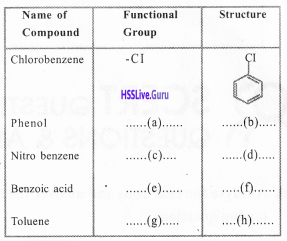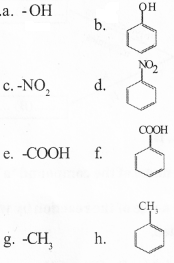Question 17.
a Write the structure of the organic comp¬ound with molecular formula QH.
b. What is the name of the compound formed when one hydrogen atom of benzene is replaced with methyl radical?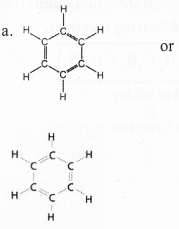b. Methyl benzene (Toluene)

Question 18.
Two equations are given below.
i. CH = CH + HCT → ……… A ………
ii. nA → B
a. Identify A and B.
b. Identify the type of reaction (i)?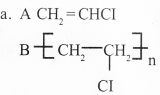Question 19.
Three equations are given below.a. Identify P, Q, R
b. Identify the name of the chemical reaction (ii) and (iii).
c. Write the IUPAC name of R.
a. P – CH2 = CH2
Q – CH3 – CH3
R – CH3 – CH2Cl
iii. Substitution Reaction
c. ChloroethaneQuestion 20.
Ethanol is an industrially important compound.
a. What is the name of 8-10% solution of ethanol?
b. How is it converted into rectified spirit?
c. What is denatured spirit?
a. Wash
b. Fractional distillation of wash
c. Product obtained by adding poisonous (methanol, pyridine) substances.

Question 21.
Uses of some important organic compounds are given. Pick out the suitable compounds from the box.
Power alcohol, Teflon, Polythene,
Ethanoic acid, Ethanol
a. For the preparation of rayon,
b. For making the coating of inner surface of non-stick cookware,
c. Solvent in paint industry,
d. As fuel in motor vehicles
a. Ethanoic acid
b. Teflon
c. Methanol
d. Power alcohol

Question 22.
Some reactants, products, and names of reactions are given in the table. Complete it.a. Substitution reaction
b. CH = CH2
c. HBr
e. H2O
f. Combustion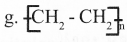h. Polymerization

Question 23.
Pick out the suitable compounds from the box for the following reactions.
CH4, C2H4, C3H8, CH3Cl
a Thermal cracking
a. C3H8
b. C2H4

### Chemical Reactions of Organic Compounds Exam Oriented Questions & Answers

Very Short Answer Type Questions (Score 1)

Question 24.
Equation of some chemical Reactions are given below. Complete it.a. A → CH3 – CH3
B → CH3 – CH2 – Cl
C → HCl
b. A → CH2 = CH2
B → CH3 – CH2 – Cl

Question 25.
Teflon used as nonstick polymer.
a. Write the structure of the monomer of this. Write the IUPAC name.
b. Write the reaction equation for the preparation of the monomer.
a. CF2 = CF2 Tetra fluroethene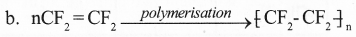Question 26.
Recognize and write A, B, C from following equation.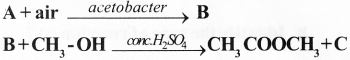A. CH3 – CH2 – OH
B. CH3 – COOH – OH
C. H2O

Short Answer Type Questions (Score 2)

Question 27.
Some chemical equations are given below. Write each type of chemical reaction.CH3 – CH = CH2 + CH3 – CH3
a. Polymerization
b. Substitution reaction
d. Combustion
e. Thermal cracking

Question 28.
Look at the following reactions on heating pentane.
i. In the absence of air
ii. In the presence of air
a. Write the name of the reaction (i), (ii).
b. Write the chemical equation for the reaction.
a. Reaction (i) – Thermal Cracking
Reaction (ii) – Combustion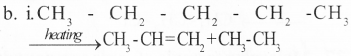ii. for writing the combustion equation for hydrocarbons we can use the equation.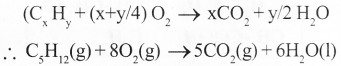Question 29.
Write each of the following.
a. Molasses
b.Wood spirit
c. Vinegar
d. Esters
a. Molasses is mother liquor left after the crystallization of sugar from sugar cane juice.
b. Poisonous chemical methanol (CH3 – OH) is known as wood spirit.
c. 5-8% ethanoic acid (CH3 – COOH) is known as vinegar.
d. esters are salts formed by the reaction ale ‘ whole and organic acids. They have the smell of fruits and flowers.

Short Answer Type Questions (Score 3)

Question 30.
Chloroform can be prepared from Methane.
a. What is the chemical formula of chlor of or m?
b. What is the name of reaction when chloroform is prepared from methane?
c. Write the chemical equation for the reaction.
a. CHCl3
b. Substitution Reaction
$$\mathrm{c.} \mathrm{CH}_{4}+\mathrm{Cl}_{2} \stackrel{\text {sunlight}}{\longrightarrow} \mathrm{CH}_{3} \mathrm{Cl}+\mathrm{HCl}$$
CH2 Cl + Cl2 → CH2Cl2 + HCl
CH2 Cl2 + Cl2 → CHCl3 + HCIQuestion 31.
Methyl Ethanoate is an ester,
a. Write the structural formula.
b. Write the structural formula of alcohol and carboxylic acid needed for the preparation.
c. Write the reaction equation for the preparation of this ester.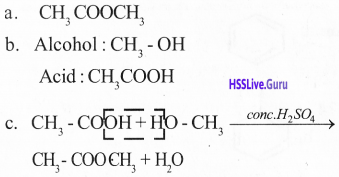Question 32.
Fill in the following.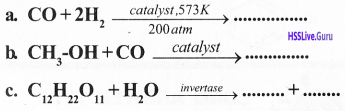Question 33.
Write one use of each of the following,
a. Methanol
b. Power alcohol
c. Butane
a. Used as solvent in the preparation of paint
b. Fuel in motor vehicle.
c. Cooking gas (LPG)

Question 34.
Write the reason for the following statements.
a. Hydrocarbons like butane are used as fuel.
b. Drinking denatured spirit is harmful
c. Esters are used in perfumes and fruit juice.
a. Combustion of hydrocarbon produces plenty of heat.
b. Ethanol on addition with poisonous material is called denatured spirit.
c. Esters have the pleasant smell of flowers and fruits.

Question 35.
Write structural formula of following com-pounds!
a. Benzene
b. Phenol
c. TolueneLong Answer Type Questions (Score 4)

Question 6.
Ethyne is a compound that belongs to the class of alkynes.
a. Write chemical formula of ethyne.
b. Write the chemical equation for the preparation of following compounds from ethyne.
i. PVC
ii. 1, 2 – dichloroethane
iii. Chloro ethane
a. CH = CH
b. i. CH = CH + HCl →Question 37.
Molecular formula of some compounds are given in the box.
C2H4 C6H14 CH3 – CH2 – CI
CH3 – COOH C6H6
a. Which is aromatic compound?
b. Which can be prepared by substitution reaction?
c. Which monomer is used in preparation of polythene?
d. Which compound can be used in food?
a. C6H6
b. CH3 – CH2 – Cl
c. C2H4
d. CH3COOH

Question 38.